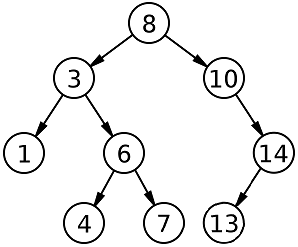New update is available. Click here to update.

# Predecessor and Successor In BST

Last Updated: 3 Dec, 2020
Difficulty: Moderate

## PROBLEM STATEMENT

#### Your task is to find the inorder successor and predecessor of the given X. Formally, print an array/list containing the inorder predecessor and successor in the same order.

##### For Example:
``````For the BST given below:
````````````The inorder predecessor of 6 is 4.
The inorder successor of 6 is 7.
The inorder predecessor of 10 is 8.
The inorder successor of 10 is 13.
``````

#### Note:

``````If there is no inorder predecessor or successor of 'X', then add -1 to the answer vector in its place.
``````
##### Input Format:
``````The first line of input contains a single integer 'T', representing the number of test cases or queries to be run.

Then the 'T' test cases follow:

The first line of each test case contains elements in the level order form.
The line consists of values of nodes separated by a single space. In case a node is null, we take -1 in its place. Refer example given below for more clarity.

The second line of each test case contains a single integer 'X'.
``````

#### Example:

``````The input for the tree depicted in the below image will be:
````````````1
2 3
4 -1 5 6
-1 7 -1 -1 -1 -1
-1 -1
``````

#### Explanation :

``````Level 1 :
The root node of the tree is 1

Level 2 :
Left child of 1 = 2
Right child of 1 = 3

Level 3 :
Left child of 2 = 4
Right child of 2 = null (-1)
Left child of 3 = 5
Right child of 3 = 6

Level 4 :
Left child of 4 = null (-1)
Right child of 4 = 7
Left child of 5 = null (-1)
Right child of 5 = null (-1)
Left child of 6 = null (-1)
Right child of 6 = null (-1)

Level 5 :
Left child of 7 = null (-1)
Right child of 7 = null (-1)

The first not-null node(of the previous level) is treated as the parent of the first two nodes of the current level. The second not-null node (of the previous level) is treated as the parent node for the next two nodes of the current level and so on.
The input ends when all nodes at the last level are null(-1).
``````
##### Note :
``````The above format was just to provide clarity on how the input is formed for a given tree.
The sequence will be put together in a single line separated by a single space. Hence, for the above-depicted tree, the input will be given as:

1 2 3 4 -1 5 6 -1 7 -1 -1 -1 -1 -1 -1
``````
##### Output Format:
``````Print a single line containing the inorder predecessor and successor of 'X' separated by a single space.

The output of each test case is printed in a separate line.
``````

#### Note:

``````You do not need to print anything, it has already been taken care of. Just implement the given function.
``````
##### Constraints:
``````1 <= T <= 5
1 <= N <= 5000
0 <= DATA <= 10^9
0 <= X <= 10^9

Where 'DATA' is the value of any node in the BST.

Time Limit: 1sec
``````## Approach 1

The main idea is to do an inorder traversal and try to find the key. If we find the key then the maximum value in the left subtree is the predecessor and the minimum value in the right subtree is the successor of the given key ‘X’.

• Maintain two variables ‘PRE’ and ‘SUC’ which point to the predecessor and successor respectively.
• We use a helper function ‘FIND_PRE_SUC_HELP’, which take the following as parameter :
• ‘ROOT’
• ‘PRE’
• ‘SUC’
• ‘KEY’ i.e. ‘X’
• If the current node's data is equal to ‘KEY’ then :
• The maximum value in the left subtree is the predecessor.
• The minimum value in the right subtree is the successor.
• If after exiting ‘FIND_PRE_SUC_HELP’ any of ‘PRE’ or ‘SUC’ are NULL then that value was not found then add -1 to the vector.
• Else add the value pointed by ‘PRE’ and then ‘SUC’.